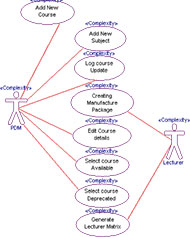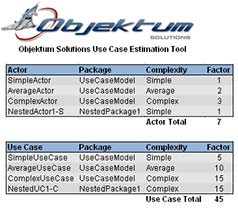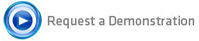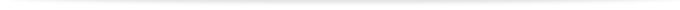Our Use Case Estimation (UCE) tool is based on Karner's Use Case Points Method, which was developed in 1993 by Gustav Karner, and provides a realistic estimate of the effort required to develop a software solution that meets the user requirements which have been expressed as a UML use case model.

This method involves the studying of the system actors and use cases, weighting them according to complexity and then applying technical and environment factors. This process follows a number of steps as follows:

1. Weight the actors
2. Weight the use cases
3. Calculate the unadjusted use case points (UUCP)
4. Determine the technical complexity factors
5. Determine the environmental factors
6. Calculate the Use Case Points (UCP)

We have developed a profile containing stereotypes and tags that allow the project estimates to be incorporated directly into the model, which can then be leverage by our tool to produce a realistic effort estimate based on the Karner Method.

Our UCE Profile provides a framework for capturing complexity and other qualitative data which is then used by our use case estimation tool to generate an effort estimate for the software development phase.  Though a continuous annotation process the following information is captured:

Weight the Actors
As the actors are developed, our profile enables them to annotate with their complexity, they are categorised as follows:

• Simple actors represents a separate entity which can be communicated with via an API. This means that interactions with the actor should be simple top implement. The multiplication factor for a simple actor is 1.
• Average actors represent an entity which communicates by a defined protocol such as TCP/IP or RS232. This represents a more complicated interaction and thus the multiplication factor is 2.
• Complex actors represent a person interacting with the system through a user interface. Obviously this interaction process is very complicated and therefore warrants the highest multiplication factor of 3.

Weight the Use CasesThe profile also enables use cases to be annotated with their level of complexity.  For each scenario we calculate the number of transactions, where a transaction can be described as the completion of a request from a primary actor.  Use cases are catergorised as:

• Simple - 3 or fewer transactions
• Average - 4 to 7 Transactions
• Complex - More than 7 transactions

Calculate the Unadjusted Use Case Points (UUCP)
The use case estimation tool automatically extracts all the above information from the model in order to calculate the unadjusted use case points (UUCP), the UUCP are calculated by using the formula

Unadjusted Use Case Points = Actor weighting + Use Case weighting

Determine the Technical Complexity Factor
Each project has differing level of complexity that can be captured directly in the model, using our UCE Profile.  Technical complexity factors include elements such as:

• Distributed system
• Response performance objectives
• End-user efficiency (online)
• Complex internal processing
• Code must be reusable
• Easy to install
• Easy to Use
• Portable
• Easy to change
• Concurrent
• Security
• Third-party access
• User training

Additional elements can be added, but each must be carefully considered and a rating of between 0 and 5 given for each, where 0 indicates that the factor is irrelevant for this particular project and 5 indicating a high importance and the fact that the item is essential in your project.

Once all the decisions have been justified our use case estimation tool calculates the Technical Complexity Factor (TFactor) for the project.

Determine the Environmental Factor
We must consider the people working within our team. They will have different strengths and experiences.This factor is called the Environmental Factor (EF).  To calculate the EF we follow a similar procedure to that discussed for the TCF.

• Familiarity with RUP
• Application Experience
• OO experience
• Lead analyst capabilities
• Motivation
• Stable requirements
• Part-time workers
• Difficult programming language

A rating of zero indicates that we have no experience in the field and a value of five means that we are experts in this area.

Once all the decisions have been justified our use case estimation tool calculates the Environmental Complexity Factor (EFactor) for the project.

Calculate the Use Case Points (UCP)
Once you have calculated the 3 factor readings (UUCP, TCF and EF) we can multiply them together to give us the use case points (UCP) for our project.

UCP = UUCP * TCF * EF

We can use this value to estimate how long the project should take to develop. The Karner process suggests a value of 20 man hours per UCP for this estimation, so to estimate project duration use this formula:

Project man-hours = 20 * UCP

With experience, this figure can be refined, personally I believe this figure should be increased when dealing with large teams as team cohesion becomes a bigger issue.

For a more accurate estimate you can add the number of environmental factors 1 to 6 that are above 3 to the number of factors in 7 and 8 that are below 3. If the total is 2 or less the figure of 20 hours is used. If the value is 3 or 4 a value of 28 should be used to calculate the project man hours.

The Use Case Estimation Tool has user-friendly, intelligent features and delivers real business value and benefits including:

• Fully integrates with Atego's Artisan Studio and IBM Rhapsody
• Shortens project estimation period and increases accuracy
• Reduces costs by allowing project managers to more intelligently allocate time and resources
• Allows estimates to be calculated even in the feasibility stage of a project
• Removes time to recalculate as a project evolves, calculations are automatically updated
• Build and estimate models to enable more accurate fee negotiation for new projects
• Time to estimate is independent of project size

### Use Case Estimation ToolContact Us

Our Use Case Estimation (UCE) tool provides a realistic estimate of the effort required to develop a software solution that meets the user requirements which have been expressed as a UML use case model.## Project Estimation tools

### Automatic Software Migration

Automatic Software Migration